EnchantedLearning.com is a user-supported site.
As a bonus, site members have access to a banner-ad-free version of the site, with print-friendly pages.

 +, - EnchantedLearning.comMath Dictionary x, ÷
 A B C D E F G H I J K L M N O P Q R S T U V W X Y ZD is for ...decagonA decagon is a ten-sided geometric figure. deciDeci is a prefix that means one-tenth. For example, a decimeter is one tenth of a meter. 1.33decimalA decimal is a base 10 number that is written with a decimal point in it. For example, 1.1, 10.43, and 0.01 are decimals.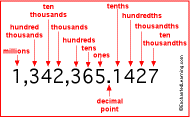decimal pointA decimal point is a dot that separates the a number into the whole number part (on the left of the decimal point) and the fraction (to the right of the decimal point). For example in 21.46, 21 is the whole number and .46 (forty-six hundredths) is the fraction.
 deficient numberA deficient number is an integer for which the sum of its proper factors (divisors) is less than the number itself. For example, 9 is a deficient number because the proper divisors of 9 are 1 and 3 which add up to 4, which is less than 9. Compare with abundant number, perfect number. degreeA degree is a measure of temperature or angle. There are 360 degrees in a circle. Each degree is divided into 60 minutes, represented by the apostrophe symbol, '. 1°=60'.denominatorThe denominator is the bottom number in a fraction. DescartesRené Descartes ( March 31, 1596 - February 11, 1650) was a French mathematician and philosopher. Descartes invented the Cartesian coordinate system and many principles of philosophy.
 diagonalA diagonal is a line that joins two non-consecutive vertices of a polygon.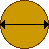diameterThe diameter is the longest distance from one side of a circle (or a sphere) to the other.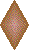diamondA diamond is a four-sided figure (a quadrilateral) whose sides are all the same length. People play baseball on a diamond-shaped field.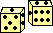diceDice are cubes with numbers on each side that are used in games. A single one is called a die.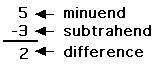differenceThe difference is the answer in a subtraction problem. digitA digit is a single numeral within a number. For example, the number 153 is a 3-digit number.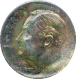dimeA dime is a coin worth ten cents.directrixThe directrix is a line that helps generate a parabola or hyperbola. For example, a parabola is a set of points (P) such that the distance from the directrix to P is equal to the distance from P to the focus F.dividendThe dividend is the number that is divided (in long division). The dividend divided by the divisor is the quotient (plus a remainder).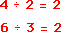divisionDivision is an operation that divides a number into portions. Any number divided by one is equal to the original number. You cannot divide any number by zero. Division is the inverse of multiplication. In long division, the dividend is divided by the divisor, resulting in a quotient plus a remainder. The ÷ symbol is called an obelus.divisorThe divisor is the number that the dividend is divided by (in long division). The dividend divided by the divisor is the quotient (plus a remainder). dodecagonA dodecagon is a twelve-sided polygon (figure).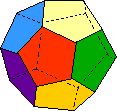dodecahedronA dodecahedron is a twelve-sided geometric solid whose faces are pentagons.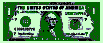dollarA dollar is worth 100 cents.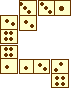dominoesDominoes is a game played with tiles that have dots on them.dotA dot is a tiny speck or point.
 ************12dozenDozen is another word for twelve.

 +, - EnchantedLearning.comMath Dictionary x, ÷
 A B C D E F G H I J K L M N O P Q R S T U V W X Y Z
 Number Line Fractions Decimals Graphs Measurement Rounding

Click on an underlined word for more information on that subject. If the math term you are looking for is not in the dictionary, please e-mail us.

Enchanted Learning®
Over 35,000 Web Pages
Sample Pages for Prospective Subscribers, or click below

 Overview of Site What's New Enchanted Learning Home Monthly Activity Calendar Books to Print Site Index K-3 Crafts K-3 Themes Little ExplorersPicture dictionary PreK/K Activities Rebus Rhymes Stories Writing Cloze Activities Essay Topics Newspaper Writing Activities Parts of Speech Fiction The Test of Time Biology Animal Printouts Biology Label Printouts Biomes Birds Butterflies Dinosaurs Food Chain Human Anatomy Mammals Plants Rainforests Sharks Whales Physical Sciences: K-12 Astronomy The Earth Geology Hurricanes Landforms Oceans Tsunami Volcano Languages Dutch French German Italian Japanese (Romaji) Portuguese Spanish Swedish Geography/History Explorers Flags Geography Inventors US History Other Topics Art and Artists Calendars College Finder Crafts Graphic Organizers Label Me! Printouts Math Music Word Wheels

## Enchanted Learning Search

 Search the Enchanted Learning website for: